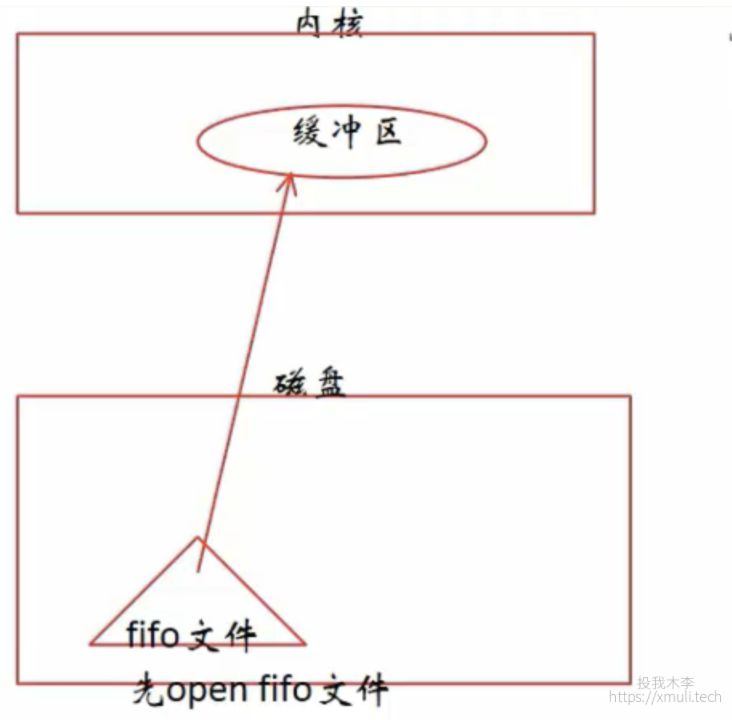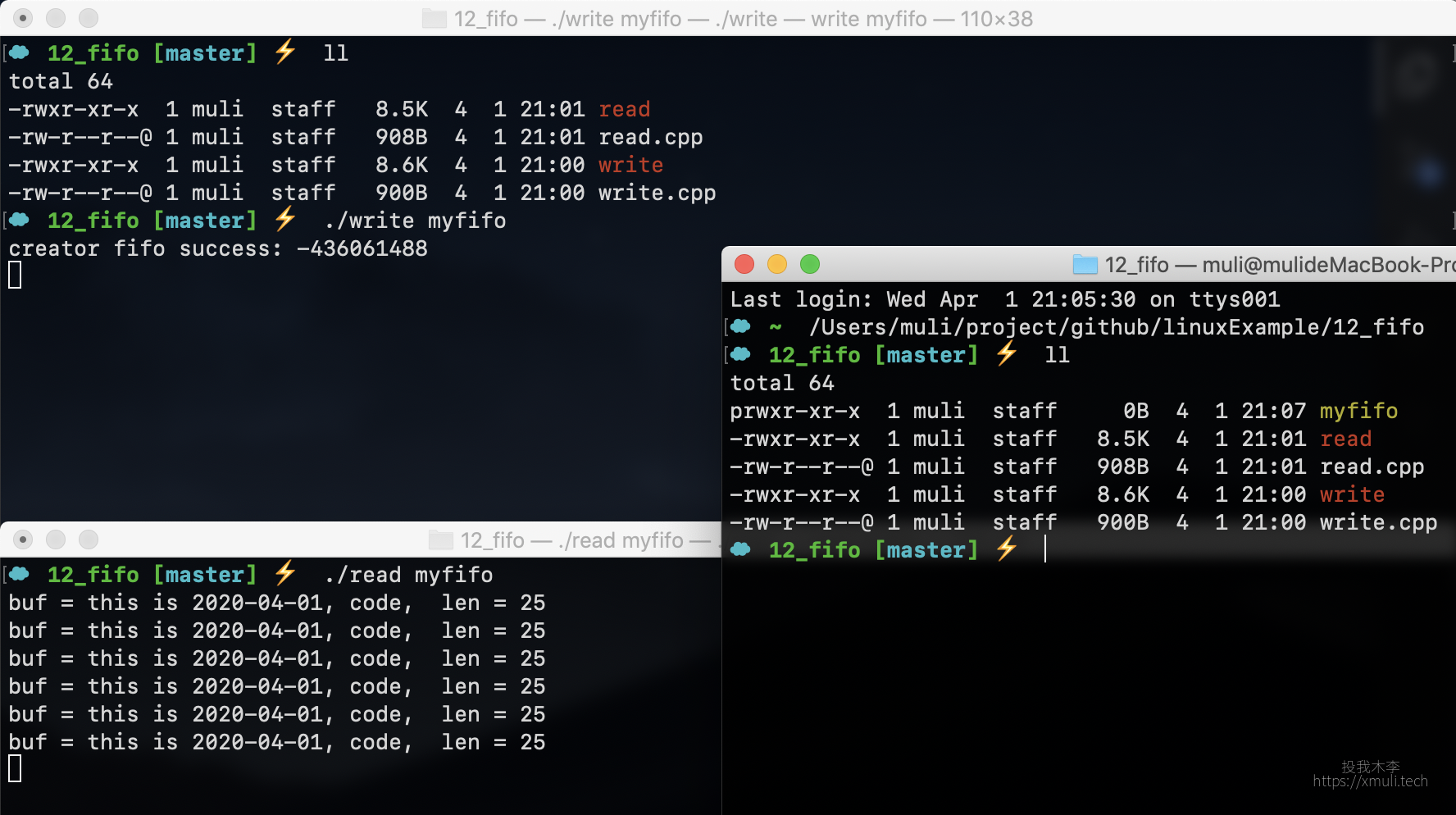简 述：　在上一篇中，写了有血缘关系的进程间的通信，使用匿名管道 `pipe`，本篇是介绍，对于无血缘关系的进程间通信，可以采用（有名）管道的 `fifo` 方式。

[TOC]

### 编程环境：

💻： `uos20` 📎 `gcc/g++ 8.3` 📎 `gdb8.0`

💻： `MacOS 10.14 ` 📎 `gcc/g++ 9.2` 📎 `gdb8.3`

### 进程间通信 IPC：

• 进程间常用的 4 种方式：
• 管道 （简单）
• 信号 （系统开销小）
• 共享映射区 （有无血缘关系的进程间通信都可以）
• 本地 socket 套接字 (稳定)

### 有名管道（fifo）：

``int fifo(int fildes);``• 本质：
• 是内核缓冲区。也是伪文件（不占用磁盘空间）
• 特点：
• 有名管道
• 在磁盘上有这样一个文件 `ls -l -> p`
• 伪文件，在磁盘大小永远为 0
• 在内核中有一个对应的缓冲区
• 使用半双工的通信方式
• 使用场景：
• 适用于没有血缘关系的进程间的通信
• 创建方式：
• 命令： mkfifo 管道名
• 函数： mkfifo()
• fifo 文件可以使用 IO 函数进行操作
• open() / close()
• 不能执行 lseek 操作

### 写一个例子：

• 代码实现：

这里写 `writ.cpp`，之后运行可执行程序 writ，当做 a 进程 ，往这个管道里面一直写数据：

``````#include <stdio.h>
#include <unistd.h>
#include <sys/wait.h>
#include <fcntl.h>
#include <sys/types.h>
#include <sys/stat.h>
#include <stdlib.h>
#include <string.h>

int main(int argc, char *argv[])
{
if (argc < 2) {
printf("请按照格式输入: ./write myfifo\n");
_exit(1);
}

int ret = access(argv, F_OK); //判断文件是否存在
if (ret == -1) {
int r = mkfifo(argv, 0777);
if (r == -1) {
perror("[creator fifo file] ");
_exit(1);
} else {
printf("creator fifo success: %d\n", argv);
}
}

int fd = open(argv, O_WRONLY);

if (fd == -1) {
perror("[open file] ");
_exit(1);
}

char const *p = "this is 2020-04-01, code";

while (true) {
//        sleep(2);  //用来验证管道阻塞这一属性的
int len = write(fd, p, strlen(p) + 1);
}

close(fd);
return 0;
}``````

这里写 `read.cpp`，之后运行可执行程序 read，当做 b 进程 ，往这个管道里面一直读数据：

``````#include <stdio.h>
#include <unistd.h>
#include <sys/wait.h>
#include <fcntl.h>
#include <sys/types.h>
#include <sys/stat.h>
#include <stdlib.h>

int main(int argc, char *argv[])
{
if (argc < 2) {
exit(1);
}

int ret = access(argv, F_OK); //判断文件是否存在
if (ret == -1) {
int r = mkfifo(argv, 0777);
if (r == -1) {
perror("[creator fifo file] ");
exit(1);
} else {
printf("creator fifo success: %d\n", argv);
}
}

int fd = open(argv, O_RDONLY);

if (fd == -1) {
perror("[open file] ");
exit(1);
}

char buf;

while (true) {
int len = read(fd, buf, sizeof(buf));
buf[len] = '\0';
printf("buf = %s,  len = %d\n", buf, len);
}

close(fd);
return 0;
}``````

• 代码分析：
为了清晰演示管道的阻塞属性，我们打开写端的 `sleep(2)` 这一行代码，故意用来制造写端的速度比读端要慢，然后观察读端的打印显示。可以清楚的得到管道是阻塞的这一结论。

• 运行效果：11_pipe